Open in App
Not now

# Genetic Algorithms for Graph Colouring | Project Idea

• Last Updated : 18 Jul, 2021

1. Project idea

In this article, we present a technique that uses Genetic Algorithms to solve the Graph Coloring Problem, and aim to find the minimum number of colors required to color a graph.

1. Check if a graph is k-colorable by finding a valid k-coloring.
2. Find the chromatic number of a graph
3. Use graph coloring to solve a Sudoku

2. Introduction

Graph coloring is an NP-Complete problem. Although a solution to an NP-Complete problem can be verified “quickly”, there is no known way to find a solution quickly. Hence, NP-Complete problems are often addressed by using approximation algorithms or heuristic methods. It is tempting, therefore, to use search heuristics like Genetic Algorithms.

Graph coloring is an assignment of labels, traditionally called “colors”, to the vertices of a graph subject to the condition that no two vertices incident with an edge is assigned the same label/color. The smallest number of colors required to color a graph G is known as its chromatic number. A coloring using at most n colors is called n-coloring. A graph that can be assigned an n-coloring is n-colorable.

The graph coloring problem is one of the most studied problems and is a very active field of research, primarily because of its application in:

• Scheduling
• Register Allocation
• Map Coloring
• Mathematical Puzzles

3. Proposed Algorithm

Graph Coloring is about minimizing the number of colors used to color the vertices of the graph. Our algorithm starts with an upper bound to the chromatic number, say k. When a valid coloring for k colors is found, we decrease k and run our algorithm again to find a valid coloring using k-1 colors. This process is repeated until it is no longer possible for our algorithm to find a valid coloring using the given number of colors. Thus we hope our algorithm will find or at least establish a tight upper bound on the chromatic number of the graph.

Algorithm: GA for Graph Coloring

Data: Adjacency Matrix of a graph G

Output: A valid k-coloring of the graph, where k = chromatic number of the graph

% best_fitness: best value of fitness encountered so far % fittest_individual: individual possessing best_fitness

% population: matrix containing all individuals of current generation % Best(P): returns the best fitness in the population P begin

```begin
generation = 0
while ( best_fitness != 0 ):
selection(population)
crossover(population)
mutation(population)
if ( Best(population) < best_fitness ):
then best_fitness = Best(population)
generation += 1
end while
return best_fitness
end```

3.1 Creating the Population

Our population comprises ‘individuals’ that are n length vectors, containing integers in the range 1 to k. Here n is the order of our graph, whereas k is the number of colors we wish to color it with. Our first-generation population is a matrix whose column entries are random numbers from 1 to k. Thus, each column corresponds to a proposed coloring of the graph albeit incorrect.

At first, k is one more than the largest degree of a vertex. This is done so, as it is the upper bound on the chromatic number of a graph.

χ(G) ≤ Δ(G) + 1

To define a fitness function, we assign a penalty of 1 for every edge that has the same colored vertices incident on it.

penalty(i, j) = 1 if there is an edge between i and j

=​ 0 Otherwise

Thus the fitness of an individual will be the sum of this penalty function over all the edges of the graph.

fitness = ∑ penalty(i, j)

3.2 Selection

This being a minimization problem, the individual having the lesser magnitude of “fitness” is the “fitter” individual.  We examine two selection algorithms for this problem. Tournament Selection and Roulette Wheel Selection.

Tournament Selection: The population is first shuffled. We group individuals in pairs (tournament size = 2), and the fitter one goes to the next generation. If our population size is N, N/2 individuals have been selected so far. We repeat this process, thereby getting N/2 more individuals, thus maintaining the population size. This technique ensures there are 2 copies of the fittest individual and no copy of the least fit individual.

Roulette-Wheel Selection: This selection is based on the relative fitness values among the individuals and probability. A “roulette wheel” is assigned chunks for each individual, the size of each chunk being proportional to the “goodness” of the individual. Then uniform random numbers are generated, analogous to spinning the wheel. The wheel is spun ( i.e. random numbers are generated ) N times, each time stopping at the “chunk” of the individual, which is selected for the next generation.

3.3 Crossover

Crossover is a recombination operator used to combine genetic information of two parents to generate new offspring. The crossover used here is Single Point Crossover. Let us show how this crossover works.

A point on both parents’ vector representation is picked randomly and designated a ‘crossover point’. Colors to the right of that point are swapped. This results in two new colorings of the graph, each carrying some genetic information from both parents.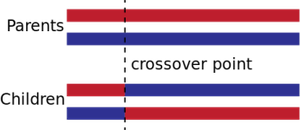3.4 Mutation

Mutation is used to maintain genetic diversity from one generation to the next. It is a small perturbation to the solution, which can help solutions stuck in local optima escape the basin of attraction. Mutation is performed quite sparingly. Thus it makes sense to perform it with some small probability, usually less than 5% or 10% . One thing that stood out while executing our algorithms was the tendency for solutions to be stuck in local optimum (as will be shown with plots in Section 4.1 ). Thus it was decided to keep this probability as high as 20%.

The mutation operator selects a vertex randomly and changes its color. This small yet effective operation is crucial to the algorithm not only arriving at a solution but also speeding up the process.

Other Details

As the algorithm evolves and since the algorithm does not know the chromatic number of the graph, χ(G), we incrementally squeeze or reduce the number of colors every time a feasible coloring with k colors is achieved. The algorithm stops either when it fails to improve the number of colors after some generations, say 10,000 generations for small graphs.

4 Experimental Results

4.1 Graph Coloring

Let us run the algorithm on a graph containing 40 vertices. We generate the adjacency matrix of such a graph by randomly allocating the entries of the matrix as 0 or 1. Our graph looks like this :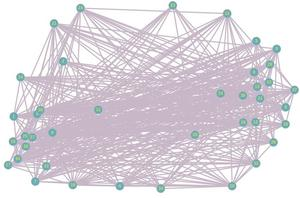The maximum degree of a vertex in this graph is 27. Hence we start with 28 colors, the upper bound on the chromatic number.

Using a population size of 200, we run our algorithm on the graph. Every 10 generations, we print the fittest individual and its fitness. For 28 colors, we have :

Generation: 10 Best_Fitness: 4 Individual: [28, 8, 25, 21, 3, 27, 16,17, 9, 28, 11, 14, 2, 13, 3, 14, 25, 12, 27, 6, 9, 14, 10, 11, 6, 23, 20, 27, 5, 24, 20, 22, 2, 26, 19, 15, 16, 18, 2, 4]

Generation: 20 Best_Fitness: 4 Individual: [19, 25, 12, 19, 11, 26, 17, 17, 9, 28, 11, 27, 24, 12, 7, 9, 25, 21, 26, 6, 9, 14, 10, 11, 6, 22, 28, 22, 12, 23, 27, 13, 8, 10, 7, 20, 16, 18, 2, 16]

Generation: 30 Best_Fitness: 0 Individual: [19, 25, 12, 19, 11, 26, 17, 17, 9, 28, 3, 26, 22, 13, 19, 4, 25, 21, 7, 9, 27, 5, 2, 23, 19, 6, 22, 10, 14, 6, 4, 1, 24, 10, 7, 20, 16, 12, 21, 21]

Using 28 colors :

Generation: 30 Best_Fitness: 0 Individual: [19, 25, 12, 19, 11, 26, 17, 17, 9, 28, 3, 26, 22, 13, 19, 4, 25, 21, 7, 9, 27, 5, 2, 23, 19, 6, 22, 10, 14, 6, 4, 1, 24, 10, 7, 20, 16, 12, 21, 21]

This is a valid coloring of the graph using at most 28 colors. The first element of the vector Individual corresponds to assigning the first vertex, the label 19, the second vertex 25, and so on. A Best_Fitness value of 0 corresponds to a valid coloring as it implies there exist no violations.

The final output of the Program is :

`The graph is 9 colorable`

We present some plots to help visualize how the fitness of the fittest individual varies with generations:

• When coloring with 22 colors :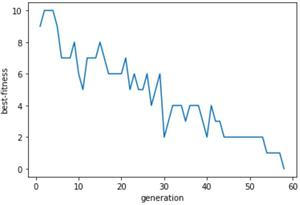Generation: 10 Best_Fitness: 6 Individual: [8, 7, 20, 14, 19, 11, 17, 17, 18, 10, 20, 3, 16, 3, 8, 13, 4, 15, 8, 4, 19, 20, 10, 20, 1, 11, 6, 1, 16, 12, 9, 8, 6, 18, 15, 9, 22, 2, 11, 21]

Generation: 20 Best_Fitness: 6 Individual: [20, 5, 22, 8, 1, 15, 4, 3, 14, 18, 5, 9, 14, 8, 20, 19, 14, 17, 3, 6, 16, 2, 21, 20, 22, 6, 10, 12, 8, 21, 1, 8, 19, 13, 3, 11, 8, 18, 11, 21]

Generation: 30 Best_Fitness: 2 Individual: [20, 5, 22, 8, 1, 15, 6, 17, 15, 18, 18, 8, 9, 1, 11, 14, 10, 5, 22, 12, 15, 20, 21, 7, 9, 17, 7, 12, 10, 21, 1, 8, 6, 13, 22, 11, 1, 2, 5, 3]

Generation: 40 Best_Fitness: 2 Individual: [16, 20, 15, 14, 19, 15, 6, 17, 15, 18, 18, 8, 9, 4, 13, 14, 10, 5, 10, 12, 22, 14, 14, 7, 9, 17, 14, 12, 10, 21, 18, 8, 6, 13, 15, 11, 1, 2, 5, 3]

Generation: 50 Best_Fitness: 2 Individual: [8, 7, 20, 12, 1, 11, 17, 3, 15, 5, 5, 9, 9, 4, 13, 14, 10, 5, 16, 19, 15, 20, 8, 7, 22, 17, 10, 22, 1, 2, 1, 8, 19, 6, 4, 8, 22, 2, 11, 18]

Using 22 colors :

Generation: 58 Best_Fitness: 0 Individual: [14, 11, 15, 14, 1,15, 17, 3, 15, 18, 5, 8, 9, 1, 13, 14, 10, 11, 10, 12, 16, 20, 8, 1, 9, 17, 21, 4, 16, 2, 1, 8, 19, 13, 4, 4, 22, 2, 11, 21]

As we approach the chromatic number of the graph, we notice an interesting trend in the above plot. At first, fitness decreases rapidly, however when it reaches close to the solution, it tends to get stuck and the fitness doesn’t improve much for a significant number of generations. Somewhat like this: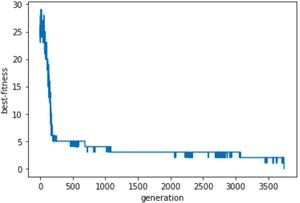To combat this, we introduce two different mutations. One which takes place in the first 200 generations, and the other which gets executed after that. The difference is in the probabilities. The former has a larger probability of mutating an Individual than the latter. This ensures that at first the search space is explored adequately fast, and at the end, only slight perturbations are made to the existing ones so that we don’t lose the “good” solutions if the converging solutions wander away.

Our program ends when it is unable to find a valid coloring using 8 colors. We allow it to run for 10000 generations. By not arriving at a valid solution in the given generations, it can be believed that there does not exist an  8-coloring for the graph, or that the value output by our algorithm is quite close to the actual chromatic number.

Generation: 9990 Best_Fitness: 4 Individual: [1, 5, 8, 5, 4, 4, 3, 6, 5, 6, 7, 3, 2, 2, 8, 1, 8, 4, 2, 5, 5, 8, 8, 1, 3, 6, 6, 4, 7, 2, 7, 2, 6, 4, 2, 1, 7, 1, 3, 3]

Generation: 10000 Best_Fitness: 4 Individual: [1, 5, 8, 5, 4, 4, 3, 6, 5, 6, 7, 3, 2, 2, 8, 1, 8, 4, 2, 5, 5, 8, 8, 1, 3, 6, 6, 4, 7, 8, 7, 2, 6, 4, 2, 1, 7, 1, 3, 3]

Using 8 colors :

Generation: 10000 Best_Fitness: 4 Individual: [1, 5, 8, 5, 4, 4, 3, 6, 5, 6, 7, 3, 2, 2, 8, 1, 8, 4, 2, 5, 5, 8, 8, 1, 3, 6, 6, 4, 7, 8, 7, 2, 6, 4, 2, 1, 7, 1, 3, 3]

Thus according to our algorithm, the graph is 9-colorable and the 9-coloring calculated is :

Using 9 colors :

Generation: 3744 Best_Fitness: 0 Individual: [8, 1, 6, 8, 5, 3, 1, 9, 8, 5, 9, 2, 2, 4, 3, 8, 3, 5, 3, 7, 1, 6, 8, 5, 6, 7, 5, 6, 4, 4, 9, 2, 7, 3, 5, 5, 4, 4, 7, 2]

5 Implementation of this problem in real life problems like Sudoku

Sudoku is a  logic-based,  combinatorial,  number-placement puzzle.  The objective is to fill a N * N grid such that every row,  column, and the N (√N * √N ) subgrids have all numbers from 1 to N. Usually, N = 9. Other variants of the puzzle also exist.

An alternate but equivalent way of presenting the rules is: Fill the grid such that no row, column, or subgrid has a repeated number. This definition makes it clear that solving a Sudoku reduces to a graph coloring problem, where we have a graph on N * N vertices. Imagine placing a vertex on an N * N grid. For every vertex, add an edge connecting the vertex to every other vertex in its row, column, and subgrid. The following image gives a visual representation for N = 9.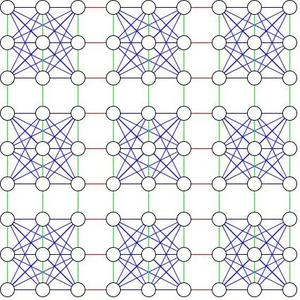The above is a graph on 81 vertices. A valid 9 coloring will represent a way to fill the Sudoku.

In order to solve Sudoku questions, we need to make a few changes. All Sudoku questions have values specified at certain locations. We will initialize all our Individuals such that they have the corresponding color(value) at their respective positions. Also, we change the mutation function to ensure these values are not mutated. Then we find a 9-coloring of the Sudoku graph2, i.e. assign labels ‘1’, ‘2’,…, ‘9’ to the nodes of the graph. The final output of the code will be a valid 9-coloring. Also, the labels specified in the input remain unchanged. An example is shown below:

• Input graph: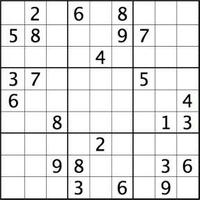On running the code, the last few lines of the output are as follows:

Generation: 144100 Best_Fitness: 2 Individual: [1, 2, 3, 6, 5, 8, 7, 4, 9, 5, 8, 4, 7, 3, 9, 7, 2, 1, 9, 6, 7, 2, 4, 1, 3, 8, 5, 3, 7, 1, 4, 9, 2, 5, 6, 8, 6, 5, 2, 1, 8, 3, 9, 7, 4, 4, 9, 8, 5, 6, 7, 2, 1, 3, 8, 3, 6, 9, 2, 4, 1, 5, 7, 2, 1, 9, 8, 7, 5, 4, 3, 6, 7, 4, 5, 3, 1, 6, 8, 9, 2]

Generation: 144120 Best_Fitness: 2 Individual: [1, 2, 3, 6, 5, 8, 7, 4, 9, 5, 8, 4, 7, 3, 9, 7, 2, 1, 9, 6, 7, 2, 4, 1, 3, 8, 5, 3, 7, 1, 4, 9, 2, 5, 6, 8, 6, 5, 2, 1, 8, 3, 9, 7, 4, 4, 9, 8, 5, 6, 7, 2, 1, 3, 8, 3, 6, 9, 2, 4, 1, 5, 7, 2, 1, 9, 8, 7, 5, 4, 3, 6, 7, 4, 5, 3, 1, 6, 8, 9, 2]

Generation: 144128 Best_Fitness: 0 Individual: [1, 2, 3, 6, 7, 8, 9, 4, 5, 5, 8, 4, 2, 3, 9, 7, 6, 1, 9, 6, 7, 1, 4, 5, 3, 2, 8, 3, 7, 2, 4, 6, 1, 5, 8, 9, 6, 9, 1, 5, 8, 3, 2, 7, 4, 4, 5, 8, 7, 9, 2, 6, 1, 3, 8, 3, 6, 9, 2, 4, 1, 5, 7, 2, 1, 9, 8, 5, 7, 4, 3, 6, 7, 4, 5, 3, 1, 6, 8, 9, 2]

The Individual having fitness 0 is a solution to the given Sudoku. When the values are entered, it looks like this:

• Output values when filled in Sudoku: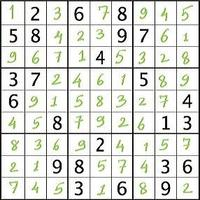6. Implementation:

## Python3

 `from` `array ``import` `*` `import` `random ` `import` `matplotlib.pyplot as plt ` `import` `numpy as np ` `Gen ``=` `np.array([]) ` `Fit ``=` `np.array([]) ` ` `  `'''Create Graph'''` `n ``=` `40` `graph ``=` `[] ` `for` `i ``in` `range``(n): ` `    ``vertex ``=` `[] ` `    ``for` `j ``in` `range``(n): ` `        ``vertex.append(random.randint(``0``, ``1``)) ` `    ``graph.append(vertex) ` `for` `i ``in` `range``(n): ` `    ``for` `j ``in` `range``(``0``, i): ` `        ``graph[i][j] ``=` `graph[j][i] ` `for` `i ``in` `range``(n): ` `    ``graph[i][i] ``=` `0` `for` `v ``in` `graph: ` `    ``print``(v) ` ` `  `'''Upper Bound for Coloring'''` `max_num_colors ``=` `1` `for` `i ``in` `range``(n): ` `    ``if` `sum``(graph[i]) > max_num_colors: ` `        ``max_num_colors ``=` `sum``(graph[i]) ``+` `1` `print``(max_num_colors) ` ` `  ` `  `'''Create Individual using given # of colors'''` `number_of_colors ``=` `max_num_colors ` `'''GA'''` `condition ``=` `True` `while``(condition ``and` `number_of_colors > ``0``): ` `    ``def` `create_individual(): ` `        ``individual ``=` `[] ` `        ``for` `i ``in` `range``(n): ` `            ``individual.append(random.randint(``1``, number_of_colors)) ` `        ``return` `individual ` `    ``'''Create Population'''` `    ``population_size ``=` `200` `    ``generation ``=` `0` `    ``population ``=` `[] ` `    ``for` `i ``in` `range``(population_size): ` `        ``individual ``=` `create_individual() ` `        ``population.append(individual) ` ` `  `    ``'''Fitness'''` `    ``def` `fitness(graph, individual): ` `        ``fitness ``=` `0` `        ``for` `i ``in` `range``(n): ` `            ``for` `j ``in` `range``(i, n): ` `                ``if``(individual[i] ``=``=` `individual[j] ``and` `graph[i][j] ``=``=` `1``): ` `                    ``fitness ``+``=` `1` `        ``return` `fitness ` ` `  `    ``'''Crossover'''` `    ``def` `crossover(parent1, parent2): ` `        ``position ``=` `random.randint(``2``, n``-``2``) ` `        ``child1 ``=` `[] ` `        ``child2 ``=` `[] ` `        ``for` `i ``in` `range``(position``+``1``): ` `            ``child1.append(parent1[i]) ` `            ``child2.append(parent2[i]) ` `        ``for` `i ``in` `range``(position``+``1``, n): ` `            ``child1.append(parent2[i]) ` `            ``child2.append(parent1[i]) ` `        ``return` `child1, child2 ` ` `  `    ``def` `mutation1(individual): ` `        ``probability ``=` `0.4` `        ``check ``=` `random.uniform(``0``, ``1``) ` `        ``if``(check <``=` `probability): ` `            ``position ``=` `random.randint(``0``, n``-``1``) ` `            ``individual[position] ``=` `random.randint(``1``, number_of_colors) ` `        ``return` `individual ` ` `  `    ``def` `mutation2(individual): ` `        ``probability ``=` `0.2` `        ``check ``=` `random.uniform(``0``, ``1``) ` `        ``if``(check <``=` `probability): ` `            ``position ``=` `random.randint(``0``, n``-``1``) ` `            ``individual[position] ``=` `random.randint(``1``, number_of_colors) ` `        ``return` `individual ` ` `  `    ``'''Tournament Selection'''` `    ``def` `tournament_selection(population): ` `        ``new_population ``=` `[] ` `        ``for` `j ``in` `range``(``2``): ` `            ``random.shuffle(population) ` `            ``for` `i ``in` `range``(``0``, population_size``-``1``, ``2``): ` `                ``if` `fitness(graph, population[i]) < fitness(graph, population[i``+``1``]): ` `                    ``new_population.append(population[i]) ` `                ``else``: ` `                    ``new_population.append(population[i``+``1``]) ` `        ``return` `new_population ` ` `  `    ``'''Roulette Wheel Selection'''` `    ``def` `roulette_wheel_selection(population): ` `        ``total_fitness ``=` `0` `        ``for` `individual ``in` `population: ` `            ``total_fitness ``+``=` `1``/``(``1``+``fitness(graph, individual)) ` `        ``cumulative_fitness ``=` `[] ` `        ``cumulative_fitness_sum ``=` `0` `        ``for` `i ``in` `range``(``len``(population)): ` `            ``cumulative_fitness_sum ``+``=` `1` `/` `\ ` `                ``(``1``+``fitness(graph, population[i]))``/``total_fitness ` `            ``cumulative_fitness.append(cumulative_fitness_sum) ` ` `  `        ``new_population ``=` `[] ` `        ``for` `i ``in` `range``(``len``(population)): ` `            ``roulette ``=` `random.uniform(``0``, ``1``) ` `            ``for` `j ``in` `range``(``len``(population)): ` `                ``if` `(roulette <``=` `cumulative_fitness[j]): ` `                    ``new_population.append(population[j]) ` `                    ``break` `        ``return` `new_population ` `    ``best_fitness ``=` `fitness(graph, population[``0``]) ` `    ``fittest_individual ``=` `population[``0``] ` `    ``gen ``=` `0` `    ``while``(best_fitness !``=` `0` `and` `gen !``=` `10000``): ` `        ``gen ``+``=` `1` `        ``population ``=` `roulette_wheel_selection(population) ` `        ``new_population ``=` `[] ` `        ``random.shuffle(population) ` `        ``for` `i ``in` `range``(``0``, population_size``-``1``, ``2``): ` `            ``child1, child2 ``=` `crossover(population[i], population[i``+``1``]) ` `            ``new_population.append(child1) ` `            ``new_population.append(child2) ` `        ``for` `individual ``in` `new_population: ` `            ``if``(gen < ``200``): ` `                ``individual ``=` `mutation1(individual) ` `            ``else``: ` `                ``individual ``=` `mutation2(individual) ` `        ``population ``=` `new_population ` `        ``best_fitness ``=` `fitness(graph, population[``0``]) ` `        ``fittest_individual ``=` `population[``0``] ` `        ``for` `individual ``in` `population: ` `            ``if``(fitness(graph, individual) < best_fitness): ` `                ``best_fitness ``=` `fitness(graph, individual) ` `                ``fittest_individual ``=` `individual ` `        ``if` `gen ``%` `10` `=``=` `0``: ` `            ``print``(``"Generation: "``, gen, ``"Best_Fitness: "``, ` `                  ``best_fitness, ``"Individual: "``, fittest_individual) ` `        ``Gen ``=` `np.append(Gen, gen) ` `        ``Fit ``=` `np.append(Fit, best_fitness) ` `    ``print``(``"Using "``, number_of_colors, ``" colors : "``) ` `    ``print``(``"Generation: "``, gen, ``"Best_Fitness: "``, ` `          ``best_fitness, ``"Individual: "``, fittest_individual) ` `    ``print``(``"\n\n"``) ` `    ``if``(best_fitness !``=` `0``): ` `        ``condition ``=` `False` `        ``print``(``"Graph is "``, number_of_colors``+``1``, ``" colorable"``) ` `    ``else``: ` `        ``Gen ``=` `np.append(Gen, gen) ` `        ``Fit ``=` `np.append(Fit, best_fitness) ` `        ``plt.plot(Gen, Fit) ` `        ``plt.xlabel(``"generation"``) ` `        ``plt.ylabel(``"best-fitness"``) ` `        ``plt.show() ` `        ``Gen ``=` `[] ` `        ``Fit ``=` `[] ` `        ``number_of_colors ``-``=` `1`

Code to generate adjacency matrix of a 9*9 Sudoku

## Python3

 `board ``=` `[] ` `for` `i ``in` `range``(``1``, ``74``, ``9``): ` `    ``row ``=` `[] ` `    ``for` `j ``in` `range``(i, i``+``9``): ` `        ``row.append(j) ` `    ``board.append(row) ` `for` `l ``in` `board: ` `    ``print``(l) ` `squares ``=` `[] ` `for` `i ``in` `range``(``1``, ``8``, ``3``): ` `    ``for` `k ``in` `range``(``1``, ``8``, ``3``): ` `        ``square ``=` `[] ` `        ``for` `j ``in` `range``(i, i``+``3``): ` `            ``for` `l ``in` `range``(k, k``+``3``): ` `                ``square.append(board[j``-``1``][l``-``1``]) ` `        ``squares.append(square) ` `for` `l ``in` `squares: ` `    ``print``(l) ` `sudoku ``=` `[] ` `for` `i ``in` `range``(``81``): ` `    ``row ``=` `[] ` `    ``for` `j ``in` `range``(``81``): ` `        ``row.append(``0``) ` `    ``sudoku.append(row) ` `for` `l ``in` `sudoku: ` `    ``print``(l) ` `Adj ``=` `[] ` `for` `i ``in` `range``(``1``, ``82``): ` `    ``row ``=` `(i``-``1``)``/``/``9` `    ``column ``=` `(i``-``1``) ``%` `9` `    ``adj ``=` `[] ` `    ``for` `j ``in` `range``(``9``): ` `        ``adj.append(board[row][j]) ` `    ``for` `j ``in` `range``(``9``): ` `        ``adj.append(board[j][column]) ` `    ``row_block ``=` `row``/``/``3` `    ``column_block ``=` `column``/``/``3` `    ``square_number ``=` `row_block``*``3` `+` `column_block ` `    ``for` `j ``in` `squares[square_number]: ` `        ``adj.append(j) ` `    ``Adj.append(adj) ` `for` `l ``in` `Adj: ` `    ``print``(l) ` `for` `i ``in` `range``(``81``): ` `    ``for` `j ``in` `Adj[i]: ` `        ``print``(i, j``-``1``) ` `        ``sudoku[i][j``-``1``] ``=` `1` `for` `i ``in` `range``(``81``): ` `    ``sudoku[i][i] ``=` `0` `for` `l ``in` `sudoku: ` `    ``print``(l) `

7. Shortcomings and Possible Remedies:

• The algorithm might be time-consuming for larger graphs(> 200 vertices). Also, the time to arrive at a solution heavily depends on the randomly initialized population.
• The stopping criterion for the algorithm is a given number of generations. This might be a poor strategy, as the algorithm might not arrive at the actual color number of the graph, given the stopping criterion. This may lead to sub-standard solutions in a few cases.
• To arrive at a valid k-coloring, the algorithm has to first find valid colorings for k+1, k+2, …. . Establishing tighter upper bounds on the chromatic number than the one mentioned in Section 3.1 can help save loads of computational time in cases where the chromatic number is far away from the aforementioned upper bound.
• As pointed out before, the algorithm has a tendency to get stuck in a local optimum, until the mutation operator perturbes it in just the right way to help it approach the solution. Using advanced mutation operators might help overcome this, which will further improve the running time.

8. Conclusion

While implementing the algorithm, we really felt as to why these algorithms are known as ‘Genetic’ Algorithms and their resemblance to Darwin’s Theory of Evolution. GAs are essentially computer simulations of nature. We saw how the fitness of the population tends to improve with iterations. The ‘survival of the fittest’ paradigm is reflected in the Selection operator. Crossover resembles reproduction, just like parents giving birth to offspring who have genetic information from both parents. The sublime simplicity of the algorithm and the impressive results that it produces show just how powerful these algorithms are.

Project Teammates:-

My Personal Notes arrow_drop_up
Related Articles